Table

When we use Table[expr, {i,imin,imax,di}] (imax-imin) doesn't have to be a
multiple of di.  See the following example.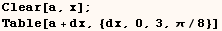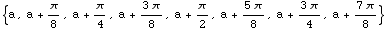You can also give Table (imax, imin,di) that are not numeric as in the next
line.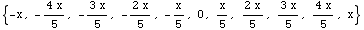However, if you want to make a long list Range, can usually make in about half the time it takes Table to do the same.   In the lines below Range is used to make the lists in two examples above.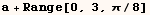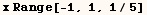The iterator in Table can be omitted if it isn't used.  The next line makes a
4x4 matrix of real numbers.  This can save considerable time.  For example
Table[Random[],{1000},{100}] evaluates in less than half the time it takes to
evaluate Table[Random[],{i,1000},{j,100}].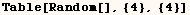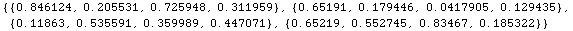The following construct can be used to make a list of lists that grow in
length.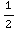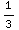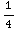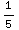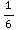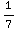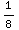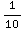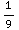Created by Mathematica  (May 16, 2004)

Back to Ted’s Tricks index page